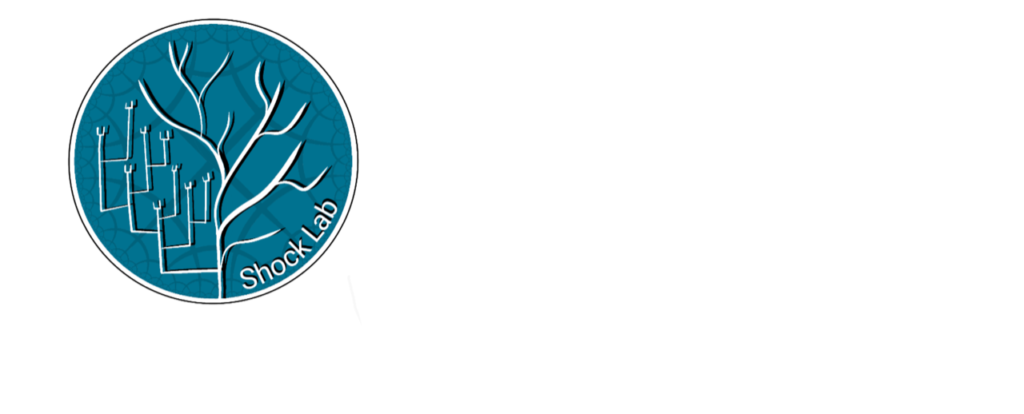ShockLab## An Introduction to Variational Inference and its Application in Deep Learning - Jacobie Mouton

### Abstract

Bayesian inference allows us to calculate the posterior distribution of unknown variables given observations, using Bayes’ Theorem. In practice however, it is typically the case that this posterior distribution is intractable to compute exactly. This tutorial introduces variational inference (VI) – which constructs an approximate posterior by framing the inference problem as an optimization problem. As part of this tutorial, we will consider tools such as Pyro which help simplify the application of VI and allow it to scale to large datasets and models. The tutorial concludes by considering an example application of VI in deep learning – Variational Autoencoders.

## SPEAKER

Jacobie Mouton is an MSc graduate from Stellenbosch University, with a thesis focused on the integration of graphical knowledge in the form of Bayesian Networks into deep learning models such as Normalizing Flows and Variational Autoencoders. Currently working at Capitec as a machine learning engineer.

## DATE

20 September 2023